﻿ Current-mode Quadrature Oscillator Using CFCCPublications are Open
Access in this journal
Article Versions
Export Article
• Normal Style
• MLA Style
• APA Style
• Chicago Style
Letter To Editor
Open Access Peer-reviewed

### Current-mode Quadrature Oscillator Using CFCC

Alok Kumar Singh, Pragati Kumar, Raj Senani
American Journal of Electrical and Electronic Engineering. 2018, 6(3), 85-89. DOI: 10.12691/ajeee-6-3-2
Receive September 02, 2018; Revised October 16, 2018; Accepted November 02, 2018

### Abstract

In this communication, a Current Follower Current Conveyor (CFCC)-based quadrature oscillator circuit has been proposed which employs a resonator-negative resistor configuration and provides oscillations in quadrature from two high impedance current output terminals of the CFCCs. The workability of the quadrature oscillator has been verified using PSPICE simulations using a CMOS CFCC implementable in 0.18 micron TSMC technology.

### 1. Introduction

A quadrature sinusoidal oscillator (QSO) circuit provides two sinusoids with 900 phase difference and finds several applications in communication and measurement systems. In communication systems they are used in quadrature mixers, single-sideband generators and direct-conversion receivers while in measurement systems such oscillators are used in vector generators or selective voltmeters (see 1 and references cited therein).

During the last two decades, many new active building blocks have been proposed in the domain of analog signal processing, a comprehensive review of which was presented in 2, wherein several new active building blocks (ABB) were also proposed. Many of the new ABBs proposed in 2 have been employed in the past to realize QSOs and other signal processing applications, which include Current Differencing Buffered Amplifier (CDBA) 3, 4, Voltage Differencing Buffered Amplifier (VDBA) 5, Voltage Differencing Transconductance Amplifier (VDTA) 6, Voltage Differencing current conveyor (VDCC) 7, Current Differencing Transconductance Amplifier (CDTA) 8, 9, 10, 11, 12, Multiple-Output Current Controlled Current Differencing Transconductance Amplifier (MO-CCCDTA) 13, Modified Current Differencing Transconductance Amplifier (MCDTA) 14, Current Follower Transconductance Amplifier (CFTA) 15, Z-copy Current Follower Transconductance Amplifier (ZC-CFTA) 16, Second-Generation Current Conveyor Transconductance Amplifier (CCII-TA) 17, Differential-Input Buffered and Transconductance Amplifier (DBTA) 18, Current-Feedback Operational Amplifier (CFOA) 19, Differential Voltage Current Conveyor (DVCC) 20, Differential Voltage Current-Controlled Conveyor Transconductance Amplifier (DVCCCTA) 21, Voltage Differencing Inverting Voltage Buffered Amplifier (VDIBA) 22, Voltage Differencing-Differential Input Buffered Amplifiers (VD-DIBA) 23, 24 and Programmable Current Amplifier 25.

It may be mentioned that while the QSOs presented in 3, 4, 5, 7, 16, 17, 18, 19, 20, 21, 22, 23, 24 operate in voltage mode (VM), those presented in 6, 8, 9, 10, 11, 12, 13, 14, 15, 16, 17, 21, 25 operate in current mode (CM). Also, of the various QSO circuits quoted above, only the circuits presented in 3, 4 and 12 offer fully-decoupled condition of oscillation and frequency of oscillation. Furthermore, out of 3, 4 and 12, the QSOs described in 3, 4 are VM oscillators while the QSO described in 12 is a CM oscillator.

It has been observed from the literature survey that a new building block named Multi-output Current Follower Current Conveyor (MO-CFCC) which was also proposed in 2 has not been utilized for the realization of QSOs so far while recently its applications in the realization of simulated impedances have been reported in 26, 27. In this letter, we propose a QSO realized with CFCC to fill this void. The workability of the proposed oscillator has been verified using PSPICE simulations in 0.18 micron TSMC technology.

### 2. CFCC-based Realization of the Current-mode Quadrature Oscillator

CFCC 2 is a five-terminal ABB with the following functionalities: the current at the z terminal is an inverted copy of the input current at ‘p’ terminal; the terminal ‘i’ tracks the potential at the terminal z and two complementary currents at the output terminals are available which are copies of the current at the ‘i’ terminal. To provide additional functionality to the CFCC, a copy of the current at the ‘z’ terminal may also be provided resulting in the Z-copy CFCC (ZC-CFCC). The symbolic representation and relations between the various port variables for this ABB are shown in Figure 1, and equation (1) respectively.

• Figure 1. Symbolic notation of the ZC-CFCC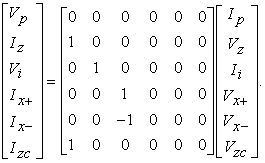(1)

The proposed current-mode quadrature oscillator circuit using CFCCs is shown in Figure 2 which consists of a parallel RLC resonator made from the CFCC along with R4, R5, C1 and C2 and a NIC-simulated negative resistor realized by the ZC-CFCC along with the resistors R1, R2 and R3.

• Figure 2. The proposed CFCC-based current mode quadrature oscillator

A straight forward routine analysis of the circuit given in Figure 2 yields the following characteristic equation (CE):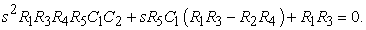(2)

From equation (2), the condition of oscillation (CO) and frequency of oscillation (FO) are found to be:

CO: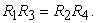(3)

FO: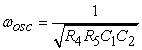(4)

However, for quadrature oscillator design, we must look into the interrelationship between I01 and I02 which is found to be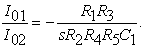(5)

Under sinusoidal steady state, equation (5) reduces to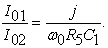(6)

The phase difference φ is, thus, equal to 90o .

### 3. Non-ideal Considerations

For a non-ideal analysis of the proposed oscillator, we have considered the various parasitic resistances and capacitances associated with the different terminals of the CFCC as follows: Rp represents the parasitic input resistance of the p port whereas Rz, Cz represent the resistance and capacitance at the z-terminal of the CFCC; similarly, Rzc and Czc represent resistance and capacitance at the zc terminal while Ri represents the output resistance of the voltage buffer of the MO-CCII (resistance looking into the i terminal); on the other hand, Rx+ and Cx+ represent output resistance and output capacitance at the x+ terminals of the CFCC and finally, Rx- and Cx- represent the output resistance and the output capacitance at the x-terminals of the CFCC. A straight forward analysis of the proposed oscillator, incorporating all the parasitic immittances described above, gives the following third order characteristic equation: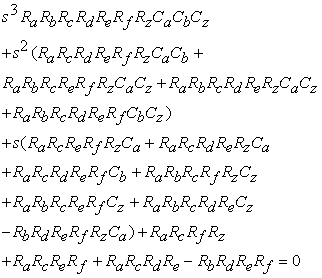(7)

where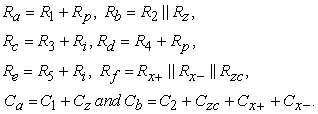We have measured the values of the various parasitic resistances and capacitances of the CFCC employed in the present work (see Figure 3) by carrying out detailed PSPICE simulations. The measured values of these non ideal parameters are found to be as summarized in Table 1.

The following approximations (ensured by selecting appropriate values of the terminating resistances and capacitances) R1 , R2, R3 ,R4 ,R5, << Rf and C1, C2>>Cx+, Cx-, Cz and Czc lead to a second order approximation of the CE from which the non-ideal CO and FO are now given by

CO: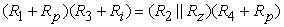(8)

FO: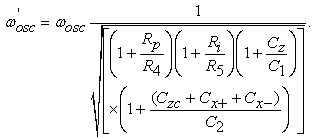(9)

The interrelationship between I01 and I02 is found to be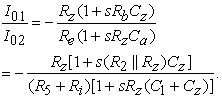(10)

The non-ideal expression of the phase difference φ is given by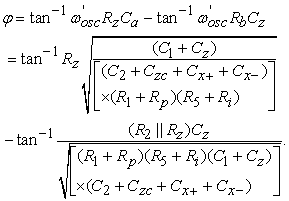(11)

Subject to the approximations used in the non-ideal analysis, it may be observed that the phase difference between the two output currents would be very close to 90o (as the angle corresponding to the argument of the first arctangent term will be close to ninety degree because of the very large value of Rz while the angle corresponding to the argument of the second arctangent term will be very small because of the very small value of Cz). We have calculated the phase difference using the values of different parasitic resistances and capacitances given in Table 1 as per equation (11) and found it to be equal to 89.52o for a design frequency of 1.59 MHz.

### 4. PSPICE Simulation Results

We now present some SPICE simulation results to demonstrate the workability of the proposed structure. The CMOS implementation of the CFCC 27 shown in Fig. 3 using 0.18 micron TSMC process technology has been used to verify the workability of the circuit presented in this paper. The values of the DC bias currents and voltages were taken as 40 µA and ± 2.5V respectively. The oscillator was designed for a frequency of 1.59 MHz by appropriately selecting the passive components as follows: C1= C2=10 pF, R1=10 kΩ, R2=10700 Ω, R3=10 kΩ, R4=10 kΩ, R5=10 kΩ. From SPICE simulations the oscillation frequency was found to be 1.50 MHz. The output waveforms are shown in Figure 4(a). The quadrature relationship of the generated waveform is indicated by the Lissajous pattern shown in Figure 4(b). The measured phase difference was found to be 90.91o. Figure 5 shows the FFT of the generated waveforms. Total harmonic distortion (THD) for current output I01 was found to be 3.2 % and for the current output I02 as 5.3 %. These simulation results thus, prove the workability of the proposed circuit.

### 5. Concluding Remarks

In this letter, a recently proposed active building block, namely, the CFCC has been used to devise a current-mode QSO. The workability of the circuit has been substantiated by SPICE simulations based on a CMOS CFCC implementable in 0.18 µm CMOS technology. The letter has, thus, added a new application of the CFCC in the area of quadrature oscillator realization, whose applications explored and known so far were only in the realization of the simulated impedances of various kinds 26, 27.

• Figure 3. An exemplary CMOS implementation of the ZC-CFCC 
• Figure 4. PSPICE Simulation Results (a) Quadrature oscillator waveforms (b) Lissajous pattern
• Figure 5. FFT of the waveforms of Io1and Io2 at 1.50 MHz

### References

Published with license by Science and Education Publishing, Copyright © 2018 Alok Kumar Singh, Pragati Kumar and Raj SenaniThis work is licensed under a Creative Commons Attribution 4.0 International License. To view a copy of this license, visit http://creativecommons.org/licenses/by/4.0/